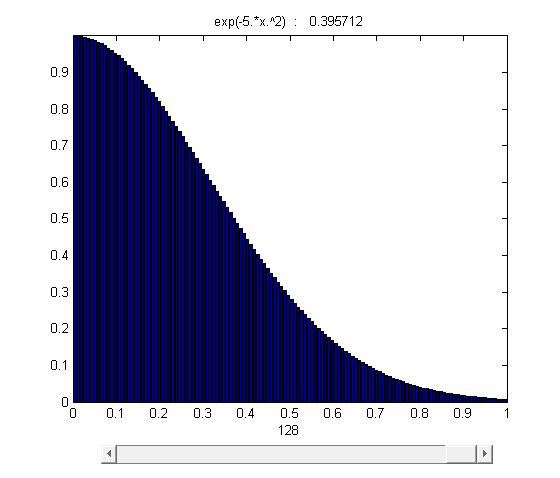# 15.1: Double and Iterated Integrals over Rectangles

$$\newcommand{\vecs}{\overset { \rightharpoonup} {\mathbf{#1}} }$$ $$\newcommand{\vecd}{\overset{-\!-\!\rightharpoonup}{\vphantom{a}\smash {#1}}}$$$$\newcommand{\id}{\mathrm{id}}$$ $$\newcommand{\Span}{\mathrm{span}}$$ $$\newcommand{\kernel}{\mathrm{null}\,}$$ $$\newcommand{\range}{\mathrm{range}\,}$$ $$\newcommand{\RealPart}{\mathrm{Re}}$$ $$\newcommand{\ImaginaryPart}{\mathrm{Im}}$$ $$\newcommand{\Argument}{\mathrm{Arg}}$$ $$\newcommand{\norm}{\| #1 \|}$$ $$\newcommand{\inner}{\langle #1, #2 \rangle}$$ $$\newcommand{\Span}{\mathrm{span}}$$ $$\newcommand{\id}{\mathrm{id}}$$ $$\newcommand{\Span}{\mathrm{span}}$$ $$\newcommand{\kernel}{\mathrm{null}\,}$$ $$\newcommand{\range}{\mathrm{range}\,}$$ $$\newcommand{\RealPart}{\mathrm{Re}}$$ $$\newcommand{\ImaginaryPart}{\mathrm{Im}}$$ $$\newcommand{\Argument}{\mathrm{Arg}}$$ $$\newcommand{\norm}{\| #1 \|}$$ $$\newcommand{\inner}{\langle #1, #2 \rangle}$$ $$\newcommand{\Span}{\mathrm{span}}$$$$\newcommand{\AA}{\unicode[.8,0]{x212B}}$$

$y=f(x)=e^{-5x^2} , 0\leq x \leq 1$

$\int_a^b f(x)\;dx = \lim_{n\rightarrow\infty}\sum_{i=1}^n f(x_i)\, \Delta x_i$

A fundamental method to calculate the area: the base of function f(x) is equally divide into n pieces whose width are $$\Delta x$$. Then $$S=\Delta x f(x_i)$$ is the area of the rectangle at the location $$\Delta x_i$$ and its height is $$f(x_i)$$ among the range $$\Delta x_i$$. Through summing all these rectangular pieces together, we can roughly estimate the area under the function $$f(x)$$ in its domain. The equation $$\sum_{i=1}^n f(x_i)\, \Delta x_i$$ can be used to represent this process.

However, $$\sum_{i=1}^n f(x_i)\, \Delta x_i$$ can only help us to estimate the value, which means errors still exist. In this case, limits help us to fix the problem.figure 15.1-1

As it was mentioned, the area was divided into n stripes. As $$n \rightarrow \infty$$ and $$\Delta x \rightarrow 0$$, stripes$$\Delta x f(x_i)$$approachs to a line whose length is equal to the height of $$f(x_i)$$. Eventually, through infinite division and accumulation, the error is reduced to zero and the sum of $$f(x) \Delta x$$ equals to the area under the curve.

$\int_a^b f(x)\;dx = \lim_{n\rightarrow\infty}\sum_{i=1}^n f(x_i)\, \Delta x_i$

Thus, we can conclude that the integral is the function of accumulation as it accumulates infinite number of strips in a certain domain to calculate the area. Similarly, the double integral is also a function of accumulation. It accumulates infinite number of small 3D strips to calculate the volume of 3D objects.

$V=\int \int_R f(x_i,y_i) dx= \lim_{n\rightarrow\infty}\sum_{k=1}^n f(x_i,y_i)\Delta A_i$

$$R$$ is the domain of the function (the area that you want to integrate over)

Explanation:as $$n \rightarrow \infty$$, the number of strips goes to infinity, $$\Delta A \rightarrow\infty$$, the error of calculation goes to 0 and the accumulation of these infinite strips eventually equals the volume of the objects.

## Theoretical discussion with descriptive elaboration

Theorem: Fubini's Theorem (First Form)

If $$f(x,y)$$ is contunuous throughout the rectangular region R: $$a\leq x \leq b, c\leq y \leq d,$$

then

$\int \int_R f(x,y)\Delta A=\int_c^d \int_a^b f(x,y)\Delta x \Delta y= \int_a^b \int_c^d f(x,y)\Delta y \Delta x.$

Fubini's Theorem is usually used to calculate the volume of three dimensional bodies

$V_i= f(x_i,y_i) \Delta A_i= f(x_i,y_i)\Delta x \Delta y$

In figure 15.1-2 , $$f(x_i,y_i)$$ is the height of the cuboid and $$\Delta A$$ is its base. $$V_i$$ means that at different location, there is a corresponding cuboid whose height is closed to the average height of the graph at the area $$\Delta A_i$$.

At the specific $$\Delta y_i$$, the cuboids with different $$\Delta x_i$$ are lined up to form a layer.

$V= \sum_{i=1}^n f(x_i,y_i) \, \Delta A_i=\sum_{i=1}^n f(x_i,y_i)\Delta x_i \Delta y_i$

As all the layers are combined together, we get a body that is approximated to the one in the next graph, but the error is still very large.

$V=\lim_{n\rightarrow \infty } \sum_{i=1}^n f(x_i,y_i) \, \Delta A_i$

Limit helps to solve this problem. As n goes to infinity, $$\Delta A_i$$ becomes smaller eventually turns to a dot. $$f(x_i, y_i) \Delta A_i$$ becomes a line and error of volume decreases to zero. Thus, the accumulation of all these lines equal to the volume.

Example 1

Now we can calculate the volume below the function $$f(x,y)=27-x^2-\frac{1}{2}y^2dx dy$$ and above $$f(x,y)$$, in the domain $$0\leq x \leq 3$$ and $$0 \leq y \leq 6$$.

\begin{align} & \int_0^6 \int_0^3 27-x^2-\frac{1}{2} y^2dx dy \\ & =\int_0^6 (27-\frac{1}{2}y^2)x-\frac{1}{3}x^3 \Big|_0^3 dy \\ & =\int_0^6 [(27-\frac{1}{2}y^2)\times3-\frac{1}{3}\times 3^3 \\ & =\int_0^6 72-\frac{3}{2}y^2 \ dy \\ & =[72y-\frac{1}{2}y^3]\Big|_0^6 \\ & = (72\times 6-\frac{1}{2}\times6^3)-(0-0) \\ & = 324 \end{align}Figure: (left) from step 1 to step 3 (right) from step 3 to step 6

Example 2

Another way to calculate the volume of the graph:

\begin{align} &\int_0^3 \int_0^6 27-x^2-\frac{1}{2} y^2dy dx \\ & = \int_0^3 (27-x^2)y-\frac{1}{6}y^3 \Big|_0^6 \ dx \\ & =\int_0^3 [(27-x^2)\times 6 -\frac{1}{6}\times6^3]-[0-0]\ dx \\ & =\int_0^3 126-6x^2\ dx \\ & = [126x-2x^3]\Big|_0^3 \\ & = (126\times3-2\times 3^3 )-(0-0) \\ & = 324. \end{align}

Reminder: the positions of $$\Delta x$$ and $$\Delta y$$ are different. So are the positions of their domains.

Example 3

Find the volume that is bounded above by the surface $$z=f(x,y)=x^2+y^2$$ and below by a rectangule R: $$0\leq x \leq 2, 0\leq y \leq 3$$.

\begin{align} & \int_0^2 \int_0^3 x^2+y^2 dy dx \\ & =\int_0^2 x^2y+\frac{1}{3}y^3 \Big|_0^3 dx \\ & =\int_0^2 3x^2+9 dx \\ & =x^3+9x\Big|_0^2 \\ & =(8+18)-0 \\ & =26 \end{align}